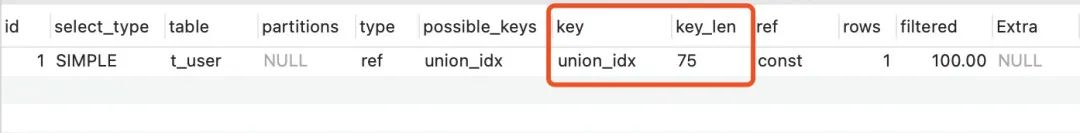# 不要再用where 1=1了！有更好的写法！

0收藏

where 1=1的作用

``````String sql  =  "select * from t_user  where 1=1 ";
if(!b.equals("")){
sql += "and  b='"+b+"'";
}   ``````

``````"select * from t_user  where and  b= 'abc' ";
``````

where 1<>1

``````create table t_temp as select * from t_user  where 1<>1
``````

where 1=1的性能问题

mysql 8.0.18，t_user表，id_no字段有索引：

``````explain select * from t_user where id_no = 'Tom25';
explain select * from t_user where 1=1 and id_no = 'Tom25';``````where 1=1的改进

``````<select id="queryUser" parameterType="com.choupangxia.entity.User" resultType="java.lang.Integer">
select count(id) from t_user u where 1=1
</if>
<if test="userNo !=null and userNo !='' ">
AND u.user_no = #{userNo}
</if>
</select>``````

``````<select id="queryUser" parameterType="com.choupangxia.entity.User" resultType="java.lang.Integer">
select count(id) from t_user u
<where>
</if>
<if test="userNo !=null and userNo !='' ">
AND u.user_no = #{userNo}
</if>
</where>
</select>``````帖子
视频
声望
粉丝
最近发布
社区精华内容